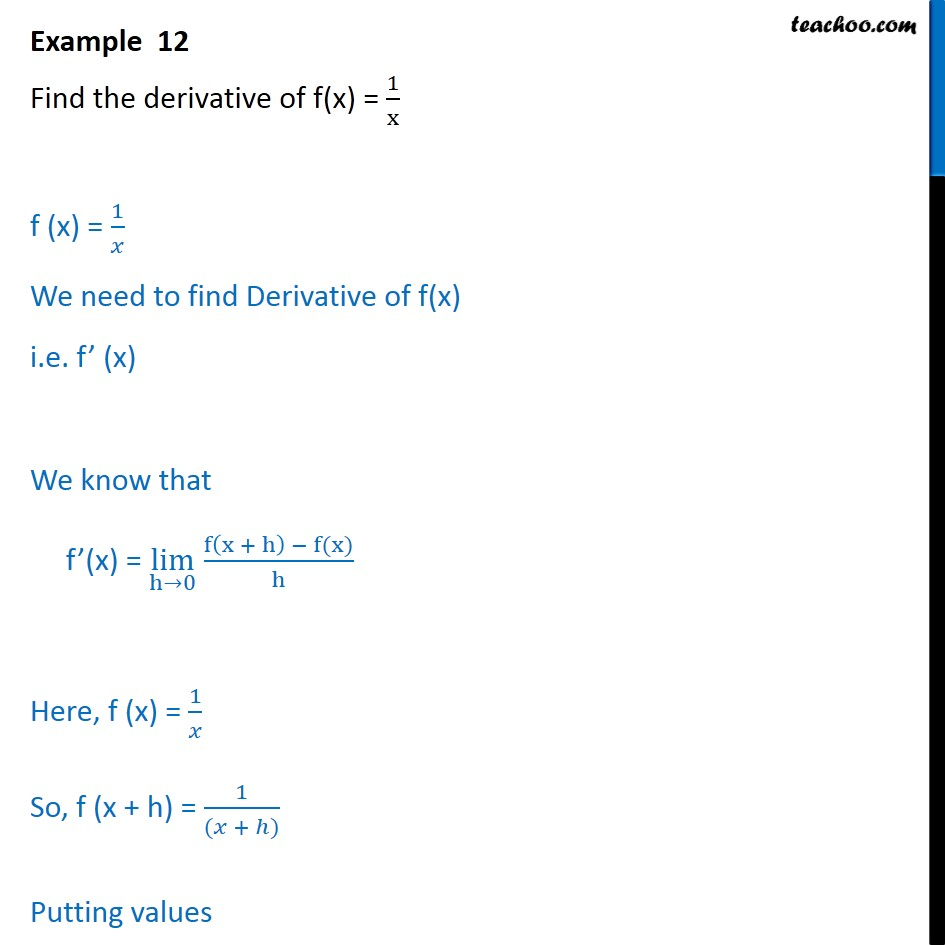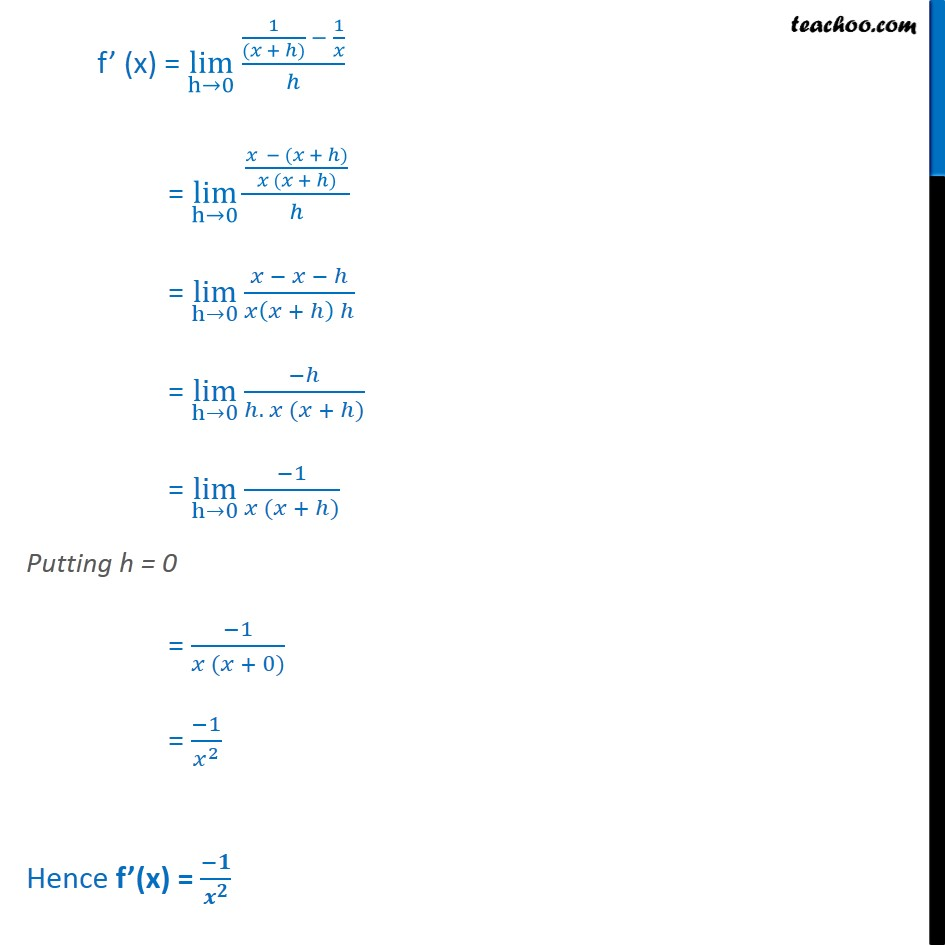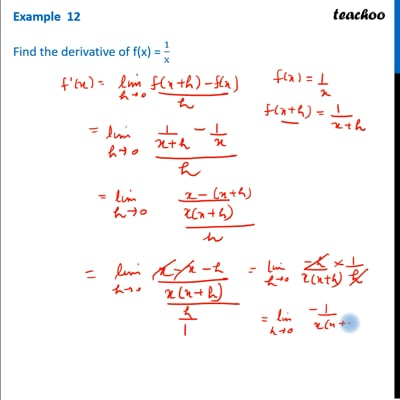Examples

Chapter 12 Class 11 Limits and Derivatives
Serial order wiseThis video is only available for Teachoo black users

Learn in your speed, with individual attention - Teachoo Maths 1-on-1 Class

### Transcript

Example 12 Find the derivative of f(x) = 1 x f (x) = 1 We need to find Derivative of f(x) i.e. f (x) We know that f (x) = lim h 0 f x + h f(x) h Here, f (x) = 1 So, f (x + h) = 1 ( + ) Putting values f (x) = lim h 0 1 ( + ) 1 = lim h 0 ( + ) ( + ) = lim h 0 + = lim h 0 . ( + ) = lim h 0 1 ( + ) Putting h = 0 = 1 ( + 0) = 1 2 Hence f (x) =